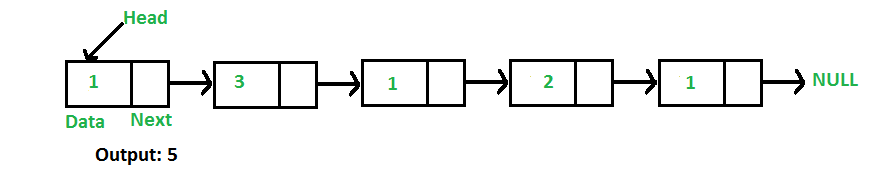# Python Program For Finding Length Of A Linked List

• Last Updated : 27 Dec, 2021

Write a function to count the number of nodes in a given singly linked list.For example, the function should return 5 for linked list 1->3->1->2->1.

Iterative Solution:

```1) Initialize count as 0
2) Initialize a node pointer, current = head.
3) Do following while current is not NULL
a) current = current -> next
b) count++;
4) Return count ```

Following is the Iterative implementation of the above algorithm to find the count of nodes in a given singly linked list.

## Python

 `# A complete working Python program to ``# find the length of a Linked List``# iteratively`` ` `# Node class``class` `Node:`` ` `    ``# Function to initialize the node object``    ``def` `__init__(``self``, data):`` ` `        ``# Assign data``        ``self``.data ``=` `data `` ` `        ``# Initialize next as null``        ``self``.``next` `=` `None` ` ` `# Linked List class contains a Node object``class` `LinkedList:`` ` `    ``# Function to initialize head``    ``def` `__init__(``self``):``        ``self``.head ``=` `None`` ` `    ``# This function is in LinkedList class. ``    ``# It inserts a new node at the beginning``    ``# of Linked List.``    ``def` `push(``self``, new_data):`` ` `        ``# 1 & 2: Allocate the Node &``        ``#        Put in the data``        ``new_node ``=` `Node(new_data)`` ` `        ``# 3. Make next of new Node as head``        ``new_node.``next` `=` `self``.head`` ` `        ``# 4. Move the head to point to the new Node``        ``self``.head ``=` `new_node`` ` `    ``# This function counts number of nodes in ``    ``# Linked List iteratively, given 'node' ``    ``# as starting node.``    ``def` `getCount(``self``):`` ` `        ``# Initialise temp``        ``temp ``=` `self``.head ``        ``count ``=` `0` `# Initialise count`` ` `        ``# Loop while end of linked list is ``        ``# not reached``        ``while` `(temp):``            ``count ``+``=` `1``            ``temp ``=` `temp.``next``        ``return` `count`` ` `# Code execution starts here``if` `__name__``=``=``'__main__'``:``    ``llist ``=` `LinkedList()``    ``llist.push(``1``)``    ``llist.push(``3``)``    ``llist.push(``1``)``    ``llist.push(``2``)``    ``llist.push(``1``)``    ``print` `(``"Count of nodes is :"``,``            ``llist.getCount())`

Output:

`count of nodes is 5`

Recursive Solution:

```int getCount(head)
1) If head is NULL, return 0.
2) Else return 1 + getCount(head->next)```

Following is the Recursive implementation of the above algorithm to find the count of nodes in a given singly linked list.

## Python

 `# A complete working Python program to ``# find the length of a Linked List ``# recursively`` ` `# Node class``class` `Node:``    ``# Function to initialize the node object``    ``def` `__init__(``self``, data):`` ` `        ``# Assign data``        ``self``.data ``=` `data  ``       ` `        ``# Initialize next as null``        ``self``.``next` `=` `None`  ` ` `# Linked List class contains a Node object``class` `LinkedList:`` ` `    ``# Function to initialize head``    ``def` `__init__(``self``):``        ``self``.head ``=` `None`` ` `    ``# This function is in LinkedList class. ``    ``# It inserts a new node at the beginning ``    ``# of Linked List.``    ``def` `push(``self``, new_data):`` ` `        ``# 1 & 2: Allocate the Node &``        ``#        Put in the data``        ``new_node ``=` `Node(new_data)`` ` `        ``# 3. Make next of new Node as head``        ``new_node.``next` `=` `self``.head`` ` `        ``# 4. Move the head to point to the new Node``        ``self``.head ``=` `new_node`` ` `    ``# This function counts number of nodes in ``    ``# Linked List recursively, given 'node' ``    ``# as starting node.``    ``def` `getCountRec(``self``, node):`` ` `        ``# Base case``        ``if` `(``not` `node): ``            ``return` `0``        ``else``:``            ``return` `1` `+` `self``.getCountRec(node.``next``)`` ` `    ``# A wrapper over getCountRec()``    ``def` `getCount(``self``):``       ``return` `self``.getCountRec(``self``.head)`` ` `# Code execution starts here``if` `__name__``=``=``'__main__'``:``    ``llist ``=` `LinkedList()``    ``llist.push(``1``)``    ``llist.push(``3``)``    ``llist.push(``1``)``    ``llist.push(``2``)``    ``llist.push(``1``)``    ``print` `(``"Count of nodes is :"``,``            ``llist.getCount())`

Output:

`Count of nodes is 5`

Please refer complete article on Find Length of a Linked List (Iterative and Recursive) for more details!

My Personal Notes arrow_drop_up